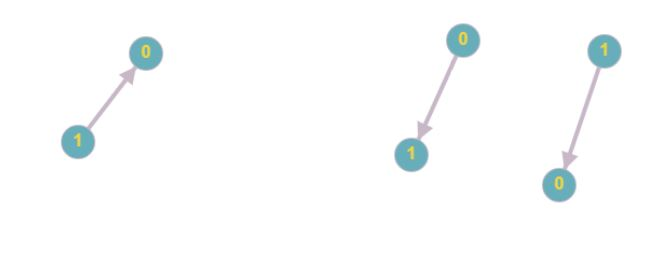Posted: 28 Mar, 2021
Difficulty: Hard

## PROBLEM STATEMENT

#### So your task is to answer each query whether it is a correct sequence or not. You will be provided with the total number of courses 'N', a list of direct prerequisite pairs, and a list of query pairs.

##### Example:``````In the image left side represents the prerequisite and on-right queries.
consider the following example suppose the number of courses ‘N = 2’prerequisites pair is [ [ 1, 0 ] ] and queries are [ [ 0, 1 ], [ 1, 0 ] ] so we return [ False, True ]  as ‘0’is not a prerequisite for the course ‘1’but ‘1’is prerequisite for the course ‘0’.
``````
##### Input Format:
``````The first line contains an integer  ‘T' which denotes the number of test cases or queries to be run. Then the test cases follow:

The first line of each test case contains, the total number of courses.

The second line of each test case contains an integer ‘M’ and ‘Q’ denoting the number of rows in the array ‘PREREQUISITE’ and ‘Q’ rows in array queries.

Then, ‘M’ lines follow:
Each line contains ‘2’ space-separated integers denoting the row values.

Then, ‘Q’ lines follow:
Each line contains ‘2’ space-separated integers denoting the row values.
``````
##### Output Format :
``````For each test case, print ‘True’ if the order is correct else ‘False’.

Print output of each test case in a separate line.
``````
##### Note:
``````You are not required to print anything explicitly. It has already been taken care of. Just implement the function.
``````
##### Constraints:
``````1 <= T <= 10^2
1 <= N <= 3*10^3
0 <= PREREQUISITE[i] <= 3*10^3

Where ‘T’ is the total number of test cases, ‘N’ is the number of courses and 'PREREQUISITE[i]' is the value of that courses.

Time Limit: 1 sec
``````Approach 1

We can think of solving this problem like a graph where we can link courses with a directed edge if the prerequisite is needed and now we can check if it is possible or not for each query. Like we make nodes with the value of courses and join them in the form of the directed graph if the prerequisite is needed.

1. First, we create a graph with the help of a prerequisite and join our nodes according to the pair given in the prerequisite
• For creating the graph we declare a matrix of size N*N where N is a number of courses.
• Now if there is a pair of prerequisites we fill that position with ‘1’which indicates there is a link between them.
• We join with the help of a directed edge.
2. Now we used a loop and check for each and every pair whether we are able to visit that node or not.
3. We used three nested for loop and if a node exists between that node we check if the shortest path is different from infinity we are able to visit that node.
4. If we are able to visit that node we return ‘True’else we return ‘False’if we are not able to visit that node and our control comes out of while statement.